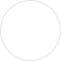J&P集团旗下公司，亚马逊,ebay,速卖通,wish,美客多,WayFair SPN服务商## 第二十一类 厨房洁具

【注释】

【2101】厨房炊事用具及容器（包括不属别类的餐具）
• 烹饪漏斗
• 分蛋器
• 烤箱用器皿
• 漏勺
• 小烤盘
• 开瓶器
• 小壶
• 非贵重金属制杯
• 烹饪用筛
• 蛋糕铲
• 烹饪擦菜板
• 芥末罐
• 厨房用砧板
• 烤面包片架
• 平底锅
• 奶壶
• 煎蛋平底锅
• 擀面杖（烹饪用）
• 筷子盒
• 贵重金属糖盒
• 厨房用漏斗
• 非电动磨咖啡器
• 烹饪用过滤器
• 柠檬榨汁器
• 焙烤盘
• 纸板杯
• 厨房用涂油脂匙
• 黄油锅
• 塑料杯
• 开胃菜用盘
• 塑料餐具垫
• 蛋糕盘
• 贵重金属碗
• 家用容器
• 碟子
• 贵重金属制蛋杯
• 开塞钻
• 蛋糕托架
• 非电动打蛋器
• 蜂蜜搅拌棒
• 比萨铲
• 水果碗
• 擦菜板（家庭用具）
• 非贵重金属制调味瓶
• 醋瓶
• 蛋糕模
• 饭铲
• 贵重金属制家用容器
• 甜点盘
• 烤肉扦
• 纸杯
• 蒸笼
• 调味瓶架
• 刀架
• 非贵重金属碟
• 油和醋用调味瓶架
• 吸管杯
• 非贵重金属制日式饭碗
• 餐用托盘
• 一次性筷子
• 日式饭碗
• 切菜板
• 非贵重金属制餐巾架
• 调味品架
• 鸣笛水壶
• 非贵重金属制糖果盒
• 非贵重金属制餐巾环
• 饮料搅拌棒
• 搅拌用碗
• 葡萄酒开瓶器
• 便当盒
• 布丁模具
• 浅碗
• 非贵重金属盘
• 三明治盒
• 手动盐研磨器
• 烤馅饼用托盘
• 蛋糕用圆顶盖
• 可重复使用的不锈钢水瓶（出售时为空的）
• 可重复使用的塑料水瓶（出售时为空的）
• 微波炉用米饭煲
• 家用非电碎蛋器
• 柑橘压汁器
• 筷枕
• 汤匙托
• 碗碟晾干架
• 制罐头用非电压力锅
• 贵重金属制餐巾架
• 贵重金属制餐巾环
• 刮锅器
• 杯形蛋糕模具
• 杯形蛋糕烘焙杯
• 可生物降解的盘子
• 一次性纸板制烤盘
• 婴儿喝水训练杯
• 寿司手工制作器
• 家用饺子模具
• 可降解的杯
• 可降解的碗
• 可降解的盘
• 煮荷包蛋器
• 树形挂杯架
• 比萨托盘支架
• 棒棒糖蛋糕用棒
• 冷冻甜点用棒
• 棒棒糖用棒
• 糕点架
• 手动饼干挤压器
• 塑料杯盘垫
• 餐具用沥水架
• 烹饪锅（非电）
• 微波炉用烹饪锅
• 碗碟托架
• 日式食案
• 蛋糕烤模
• 露营用烤架
• 贵重金属制调味瓶架
• 贵重金属制分隔层饰盘
• 餐桌用洗指碗
• 玻璃制水果碗
• 煎锅（非电动）
• 日式叠层食盒
• 贵重金属制日式饭碗
• 厨房用瓮（非贵重金属）
• 午餐盒（煮饭用餐具盒）
• 搅拌用杯
• 户外使用的便携式烹饪套具
• 煮饭锅（非电动）
• 色拉脱水器
• 上菜用的碗
• 烤比萨用石板
• 贵重金属制盐瓶
• 水壶（非贵重金属制）
• 贵重金属糖罐
• 饮料杯用隔热杯托
• 金属制饭盒
• 塑料制饭盒
• 旋转式奶酪擦丝器
• 非电绞肉机
• 压薯器
• 家用非电食品搅拌器
• 非电牛奶起泡器
• 家用非电食品搅拌机
• 便携式饮料容器夹
• 水瓶（空）
• 分成九格的漆器餐盘
• 野营用锅碗
• 食物用滴油管
• 非电烤盘（烹饪用具）
• 可生物降解的碗
• 可生物降解的杯
• 可生物降解的托盘
• 可堆肥的托盘
• 烹饪用平底锅（非电）
• 贵重金属制调味瓶
• 非电烹饪蒸锅
• 家用奶酪刨丝器
• 冰块铲勺（酒吧器具）
• 铲勺（家用或厨房用具）
• 家用金属篮
• 咖啡铲勺
• 出售时为空的摇杯
• 成套的烹饪锅
• 黄油碟
• 黄油碟罩
• 开塞钻（电或非电）
• 开瓶器（电或非电）
• 调味瓶
• 菜单卡片夹
• 炖锅
• 鸡尾酒调酒器
• 家用过滤器
• 锅盖
• 烹饪锅
• 烹饪用模具
• 厨房用切菜板
• 漏斗
• 煎锅
• 午餐盒
• 烤架（烹饪用具）
• 面包屑盘
• 蛋杯
• 切面包板
• 纸盘
• 馅饼用铲
• 手动胡椒研磨器
• 胡椒瓶
• 厨房用擦菜板
• 家用或厨房用容器
• 餐巾环
• 色拉碗
• 盐瓶
• 餐巾架
• 糖碗
• 分隔层饰盘
• 烹饪用非电高压锅
• 奶瓶用非电加热器
• 糖果盒
• 非电烧水壶
• 非电油炸锅
• 奶酪盘罩
• 家用篮
• 家用托盘
• 碟罩
• 水瓶
• 水壶
• 厨房容器
• 长颈瓶
• 烘蛋奶饼的非电铁模
• 面包箱
• 筷子
• 鸡尾酒搅拌棒
• 饼干模具
• 饼干筒
• 非电加热的火锅
• 餐桌用旋转盘
• 饭盒
• 切糕点器
• 一次性盘子
• 烘焙垫
• 隔热垫
• 家用非电鸡蛋分离器
• 冰块夹
• 色拉夹
• 上菜勺
• 厨房用杵
• 厨房用研钵
• 冰淇淋挖勺
• 胡桃钳
• 方糖钳
• 盛葡萄酒用长柄勺
• 铁锅
（备注）